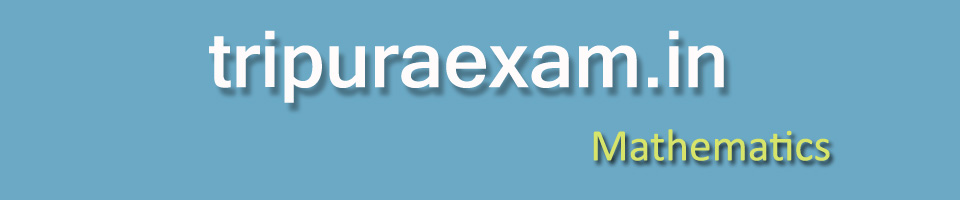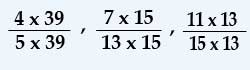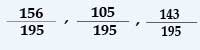Welcome, Guest

## SOLVED PROBLEM

(TPSC - Small Savings held on 4th April,2010)

Q1 A's salary is 20% below of B's salary. By how much percent is B's salary is above A's ?

Sol : Let B's salary is 100.
Therefore, A's salary is 80.
Therefore, B's salary is above A's salary is 2080 X 100%
= 25%
Ans : = 25%

Q2. P's money is Q's money as 4 : 5 and Q's money is R's money as 2 : 3. If P has 800, R has

Sol : P:Q = 4 : 5

PQ = 45 (Given P = 800)
800Q = 45
⇒ Q = 5 x 8004 = 1000

Again, Q : P = 2 : 3

QP = 23 (Here Q = 1000)
1000P = 23
⇒ P = 3 x 10002 = 1500
Ans: P = 1500

Q3. At what rate percent per year simple interest will a sum be doubled in 8 years?

Sol: Let, Principal is 100 , therefore after 8 years a sum 200 i.e. simple interest 100.
In 8 years 100 rupees simple interest 100

In 1 years 100 rupees simple interest 1008
= 12 48
= 12 12
Ans: 1212

Q4. A tap can fill in 25 minutes and another tap can empty in 50 minutes. If both are opened simultaneously, when will be the tank full?

Sol : Fill in 25 minutes i.e. 100% but same time empty will be 50% as the time taken double to empty.

So, remain in tank 50%. To fill more 50% more 25 minutes time required.

i.e. total time 25 + 25 minutes = 50 minutes.
Ans : 50 minutes.

Q5. The cost of paving a rectangular courtyard of 30 m long  and 20 m wide with tiles of 12 cm long and 10 cm wide at Rs. 40 per thousand tiles is

Sol : 30 m = 30 x 100 cm = 3000 cm and 20 m = 20 x 100 cm = 2000 cm
Area of courtyard = length x bredth
= 3000 x 2000 sq. cm
= 6000000 sq. cm
Area of tiles = length x bredth
= 12 x 10 sq. cm
= 120 sq. cm

Total tiles required = Area of courtyard ÷ Area of tiles
= 6000000120 Nos.

= 50000 nos.
Cost per thousand is Rs. 40
Here 50 thousand tiles require
Therefore, Total cost  50 x 40  = 2000
Ans : 2000

Q6. In a cricket match, average number of runs scored by five players comes to 25. One of them scored 13 rans. What is the average score of remaining players?

Sol : Total run of five players = 25 x 5 = 125
One scored 13, so remaining four’s total run = 125 – 13 = 112

Therefore, average run = 1124 = 28
Ans : 28

Q7. Which of the following is the greatest?

45 , 713 , 1115

Sol: Here LCM is 195, So, multiply by other factor to get denominator 195  and by the the same factor multiply the numerator.i.e 45 is greatest.

More..